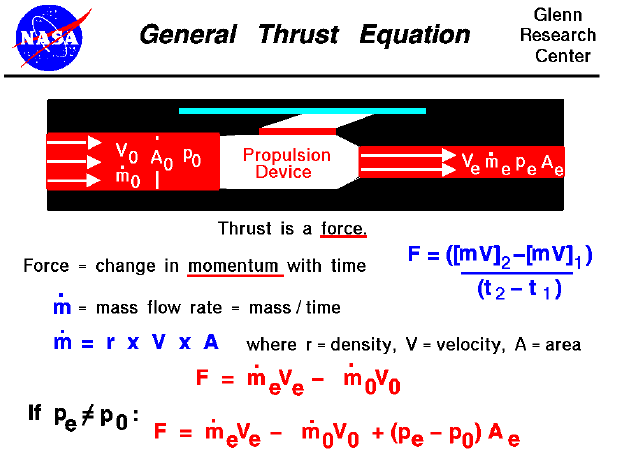Thrust is the force which moves an aircraft through the air. Thrust is generated by the engines of the airplane. How is thrust generated?

Thrust is a mechanical force. It is generated through the reaction of accelerating a mass of gas. The gas is accelerated to the the rear and the engine (and aircraft) is accelerated in the opposite direction. To accelerate the gas, we need some kind of propulsion system. We will discuss the details of various propulsion systems on some other pages. For right now, let us just think of the propulsion system as some machine which accelerates a gas.

From Newton's second law of motion, we can define a force (F) to be the change in momentum of an object with a change in time (t). Momentum is the object's mass (m) times the velocity (V). So, between two times (t1) and (t2), the force is given by:

F = ((m * V)2 - (m * V)1) / (t2 - t1)

If we keep the mass constant and just change the velocity with time we obtain the simple force equation - force equals mass time acceleration (a)

F = m * a

If we are dealing with a solid, keeping track of the mass is relatively easy; the molecules of a solid are closely bound to each other and a solid will retain its shape. But if we are dealing with a fluid (liquid or gas) and particularly if we are dealing with a moving fluid, keeping track of the mass gets tricky. For a moving fluid, the important parameter is the mass flow rate. Mass flow rate is the amount of mass moving through a given plane over some amount of time. Its dimensions are mass/time (kg/sec, slug/sec, ...) and it is equal to the density (r) times the velocity (V) times the area (A). Aerodynamicists often denote this parameter as "m dot" (m with a little dot over the top). Note that "m dot" is not simply the mass of the fluid, but is defined to be the mass flow rate, the mass per unit time

m dot = r * V * A

Since the mass flow rate already contains the time dependence (mass/time), we can express the change in momentum across the propulsion device as the change in the mass flow rate times the velocity. We will denote the exit of the device as station "e" and the free stream as station "0". Then

F = (m dot * V)e - (m dot * V)0

A units check shows that we are dealing with mass/time x length/time = mass x length/ time squared. This is the dimension of a force. There is an additional effect which we must account for if the exit pressure (p) is different from the free stream pressure. The fluid pressure is related to the momentum of the gas molecules and acts perpendicular to any boundary which we impose. If there is a net change of pressure in the flow there is an additional change in momentum. Across the exit area we may encounter an additional force term equal to the exit area (Ae) times the exit pressure minus the free stream pressure. The most general thrust equation is then given by:

F = (m dot * V)e - (m dot * V)0 + (pe - p0) * Ae

Normally, the magnitude of the pressure-area term is small relative to the m dot-V terms. The nozzle of a jet engine is usually designed to make the exit pressure equal to free stream. In that case, the pressure term in the general equation is equal to zero and we can use the previous thrust equation. The force (thrust) is equal to the exit mass flow rate times the exit velocity minus the free stream mass flow rate times the free stream velocity. Since the exit mass flow rate is nearly equal to the free stream mass flow rate, and the free stream is all air, we can call the mass flow rate through the engine the engine airflow rate.

Looking at the thrust equation very carefully, we see that there are two possible ways to produce high thrust. One way is to produce high thrust is to make the engine airflow rate as high as possible. As long as the exit velocity is greater than the free stream (entrance) velocity, a high engine airflow will produce high thrust. This is the design theory behind propeller aircraft and high-bypass turbofan engines. A large amount of air is processed each second, but the air velocity is not changed very much. The other way to produce high thrust is to make the exit velocity very much greater than the incoming velocity. This is the design theory behind pure turbojets and turbojets with afterburners. A moderate amount of airflow is accelerated to a high velocity in these engines. If the exit velocity becomes very high, there are other physical processes which become important and affect the efficiency of the engine. These effects are described in detail on another page.

For rocket engines, because the oxidizer is carried on board, the free stream mass flow rate is zero. So all of the thrust of a rocket engine is produced by the exit mass flow rate times the exit velocity. For gas turbine engines, we can absorb the engine airflow dependence into a more useful parameter called the specific thrust. For both rockets and turbojets, the nozzle performs two important roles. The design of the nozzle determines the exit velocity for a given pressure and temperature. And because of flow choking in the throat of the nozzle, the nozzle design also sets the mass flow rate through the propulsion system. Therefore, the nozzle design determines the thrust of the propulsion system as defined on this page. You can investigate nozzle operation with our interactive nozzle simulator.

Guided Tours